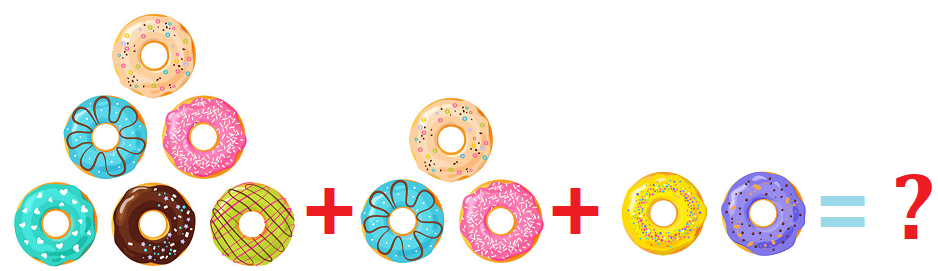# Math > Year 2

## Adding and subtracting three 1-digit numbers

What are one-digit numbers?
One-digit numbers are the numbers made up of only one digit i.e., ones.
For example, 1, 2, 3 etc.

2 + 4 + 1

2 + 4 = 6
6 +  1 = 7
So, 2 + 4 + 1 = 7

Changing order

For adding 3 one-digit numbers, we can change the order of the numbers.
For example:

(3 + 1 + 2) = (1 + 2 + 3) = (3 + 2 + 1)

This is because addition is commutative. This means that changing the order of numbers in the addition question doesn’t change the answer.

Example: Henry has 4 sweets. His brother gave him 1 sweet and his sister gave him 2 sweets. How many sweets does Henry have now?
4 sweets + 1 sweet + 2 sweets = 5 sweets + 2 sweets = 7 sweets

Let's try more questions.

Question 1:Count the number of doughnuts.

6 + 3 + 2

= 9 + 2 = 11

Question 2:
Dacie has one cat in the house. He bought 2 more cats and 3 dogs. What is the total number of pets in the house?

Number of cats = 1

Number of cats bought = 2

Number of dogs = 3

Total number of pets = 1 + 2 + 3 = 6

//